# 16.10: Preparative Methods for Aldehydes and Ketones

$$\newcommand{\vecs}{\overset { \rightharpoonup} {\mathbf{#1}} }$$ $$\newcommand{\vecd}{\overset{-\!-\!\rightharpoonup}{\vphantom{a}\smash {#1}}}$$$$\newcommand{\id}{\mathrm{id}}$$ $$\newcommand{\Span}{\mathrm{span}}$$ $$\newcommand{\kernel}{\mathrm{null}\,}$$ $$\newcommand{\range}{\mathrm{range}\,}$$ $$\newcommand{\RealPart}{\mathrm{Re}}$$ $$\newcommand{\ImaginaryPart}{\mathrm{Im}}$$ $$\newcommand{\Argument}{\mathrm{Arg}}$$ $$\newcommand{\norm}{\| #1 \|}$$ $$\newcommand{\inner}{\langle #1, #2 \rangle}$$ $$\newcommand{\Span}{\mathrm{span}}$$ $$\newcommand{\id}{\mathrm{id}}$$ $$\newcommand{\Span}{\mathrm{span}}$$ $$\newcommand{\kernel}{\mathrm{null}\,}$$ $$\newcommand{\range}{\mathrm{range}\,}$$ $$\newcommand{\RealPart}{\mathrm{Re}}$$ $$\newcommand{\ImaginaryPart}{\mathrm{Im}}$$ $$\newcommand{\Argument}{\mathrm{Arg}}$$ $$\newcommand{\norm}{\| #1 \|}$$ $$\newcommand{\inner}{\langle #1, #2 \rangle}$$ $$\newcommand{\Span}{\mathrm{span}}$$

A number of useful reactions for the preparation of aldehydes and ketones, such as ozonization of alkenes and hydration of alkynes, have been considered in previous chapters. These and other methods of preparation are summarized in Tables 16-7 and 16-8 at the end of the chapter. Only a few rather general methods that we have not discussed will be taken up here.

## Oxidation of 1,2-Diols and Alkenes

Aldehydes and ketones often can be prepared by oxidation of alkenes to 1,2-diols (Sections 11-7C and 11-7D), followed by oxidative cleavage of the 1,2-diols with lead tetraethanoate or sodium periodate. For example,Cleavage of glycols with these reagents proceeds according to the following stoichiometry:## Oxidation of Primary Alcohols and Related Compounds

In Chapter 15 primary alcohols, $$\ce{RCH_2OH}$$, were shown to be readily oxidized to aldehydes, $$\ce{RCHO}$$, and secondary alcohols, $$\ce{R_2CHOH}$$, to ketones, $$\ce{R_2CO}$$, by inorganic reagents such as $$\ce{CrO_3}$$ and $$\ce{KMnO_4}$$. However, it is a problem to avoid overoxidation with primary alcohols because of the ease with which aldehydes are oxidized to acids, $$\ce{RCHO} \rightarrow \ce{RCO_2H}$$. A milder oxidant is methylsulfinylmethane [dimethyl sulfoxide, $$\ce{(CH_3)_2S=O}$$], and this reagent can be used to prepare aldehydes from alcohols by way of an intermediate such as the ester or halide in which the $$\ce{OH}$$ group is converted to a better leaving group: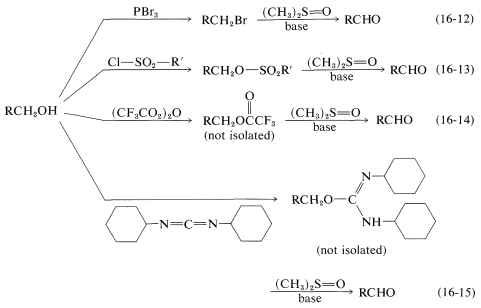Whichever method is employed, the key step is the formation of an alkoxysulfonium salt, $$7$$, by a displacement reaction involving dimethyl sulfoxide as an oxygen nucleophile. (Notice that the $$\ce{S=O}$$ bond, like the $$\ce{C=O}$$ bonds, is strongly polarized as $$\overset{\oplus}{\ce{S}} \ce{-} \overset{\ominus}{\ce{O}}$$.)In the examples listed in Equations 16-12 through 16-15, the $$\ce{X}$$ group is $$\ce{Br}$$, $$\ce{-OSO_2R'}$$, $$\ce{-O_2CCF_3}$$, and, respectively. In the nest step a sulfur ylide, $$8$$, is formed from the reaction of a base with $$7$$, but the ylide evidently is unstable and fragments by an internal $$E2$$ reaction to form an aldehyde:## Reduction of Carboxylic Acids to Aldehydes

Conversion of a carboxylic acid to an aldehyde by direct reduction is not easy to achieve, because acids generally are difficult to reduce, whereas aldehydes are easily reduced. Thus the problem is to keep the reaction from going too far.

The most useful procedures involve conversion of the acid to a derivative that either is more easily reduced than an aldehyde, or else is reduced to a substance from which the aldehyde can be generated. The so-called Rosenmund reduction involves the first of these schemes; in this procedure, the acid is converted to an acyl chloride, which is reduced with hydrogen over a palladium catalyst to the aldehyde in yields up to $$90\%$$. The rate of reduction of the aldehyde to the corresponding alcohol is kept at a low level by poisoning the catalyst with suflur: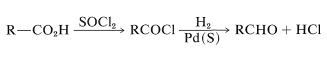Metal hydrides, such as lithium aluminum hydride, also can be used to reduce derivatives of carboxylic acids (such as amids and nitriles see Table 16-6) to aldehydes. An example follows:## Rearrangements of 1,2-Diols

Many carbonyl compounds can be synthesized by acid-catalyzed rearrangements of 1,2-diols (a type of reaction often called the "pinacol-pinacolone" rearrangement).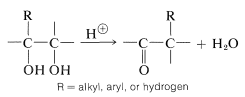The general characteristics of the reaction are similar to those of carbocation rearrangements (Section 8-9B). The acid assists the reaction by protonating one of the $$\ce{-OH}$$ groups to make it a better leaving group. The carbocation that results then can undergo rearrangement by shift of the neighboring $$\ce{R}$$ group with its pair of bonding electrions to give a new, thermodynamically more stable species with a carbon-oxygen double bond (see Section 16-7).

The prototype of this rearrangement is the conversion of pinacol to pinacolone as follows:## Rearrangements of Hydroperoxides

An important method of preparing carbonyl (and hydroxy) compounds, especially on an industrial scale, is through rearrangements of alkyl hydroperoxides:The peroxides can be made in some cases by direct air oxidation of hydrocarbons,and in others by sulfuric acid-induced addition of hydrogen peroxide (as $$\ce{H-O_2H}$$) to double bonds:(Notice that hydrogen peroxide in methanoic acid behaves differently toward alkenes in producing addition of $$\ce{HO-OH}$$, Section 11-7D.) The direct air oxidation of hydrocarbons is mechanistically similar to that of benzenecarbaldehyde (Section 16-7).

The rearrangements of hydroperoxides are acid-catalyzed and are analogous to carbocation rearrangements except that positive oxygen (with only six valence electrons) instead of positive carbon is involved in the intermediate stage: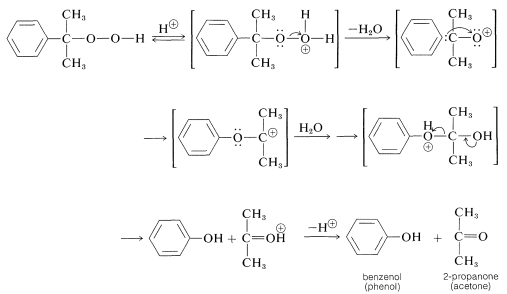In principle, either phenyl or methyl could migrate to the positive oxygen, but only phenyl migration occurs in this case. The rearrangement reaction is closely related to the Baeyer-Villiger reaction (Section 16-7).

## Aldehydes by Hydroformylation of Alkenes

This reaction is important for a number of reasons. It is an industrial synthesis of aldehydes from alkenes by the addition of carbon monoxide and hydrogen in the presence of a cobalt catalyst. A prime example is the synthesis of butanal from propene, in which 2-methylpropanal also is formed:As you can see, the reaction formally amounts to the addition of methanal as $$\ce{H-CHO}$$ to the alkene double bond. Because one additional carbon atom is introduced as a "formyl" $$\ce{CHO}$$ group, the reaction often is called hydroformylation, although the older name, oxo reaction, is widely used.

Hydroformylation to produce aldehydes is the first step in an important industrial route to alcohols. The intermediate aldehydes are reduced to alcohols by catalytic hydrogenation. Large quantities of $$\ce{C_4}$$-$$\ce{C_8}$$ alcohols are prepared by this sequence:The history of the oxo reaction is also noteworthy. It was developed originally in Germany in the years following World War I. At that time, the German chemical industry was faced with inadequate supplies of petroleum. Many German chemists therefore turned to research on ways by which hydrocarbons could be synthesized from smaller building blocks, particularly carbon monoxide and hydrogen derived from coal. The success achieved was remarkable and led to alkane and alkene syntheses known as the Fischer-Tropsch process: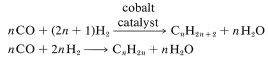​​​​​​

This reaction in turn led to the discovery that aldehydes were formed by the further addition of carbon monoxide and hydrogen to alkenes, and was further developed as the oxo process for production of alcohols. The combination $$\ce{CO} + \ce{H_2}$$ often is called "synthetic gas". It is prepared by the reduction of water under pressure and at elevated temperatures by carbon (usually coke), methane, or higher-molecular-weight hydrocarbons: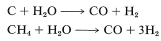## Carbonylation of Alkylboranes

The aldehyde synthesis by hydroformylation of alkenes described in the preceding section can be achieved indirectly using boron hydrides. An oversimplified expression of this reaction isThe overall reaction is quite complex but involves a rearrangement similar to that described for the hydroboration-oxidation of alkenes (Section 11-6E). The first step is hydroboration of the alkene to a trialkylborane. When the trialkylborane is exposed to carbon monoxide, it reacts (carbonylates) to form a tetracovalent boron, $$9$$:The complex $$9$$ is unstable and rearranges by transfer of an alkyl group from boron to the electron-deficient carbonyl carbon to give $$10$$: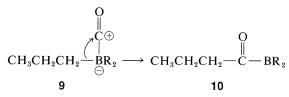Now, if a metal-hydride reducing agent, such as $$\ce{LiAlH_4}$$, is present, the carbonyl group of $$10$$ is reduced and $$11$$ is formed:The reduction product, $$11$$, can be converted to an aldehyde by oxidation with aqueous hydrogen peroxide, provided the pH is carefully controlled. (Remember, aldehydes are unstable in strong base.)You may have noticed that only one of the three alkyl groups of a trialkylborane is converted to an aldehyde by the carbonylation-reduction-oxidation sequence. To ensure that carbonylation takes the desired course without wasting the starting alkene, hydroboration is achieved conveniently with a hindered borane, such as "9-BBN", $$12$$. With $$12$$, only the least-hindered alkyl group rearranges in the carbonylation step:Carbonylation of alkylboranes also can produce ketones. The conditions are similar to those in the aldehyde synthesis except that the hydride reducing agent is omitted. By omitting the reducing agent, a second boron-to-carbon rearrangement can occur. Oxidation then produces a ketone:Rearrangement will continue a third time (ultimately to produce a tertiary alcohol) unless movement of the alkyl group remaining on boron in $$13$$ is prevented by steric hindrance.